# IBM of clonal plant dynamicsAn Individual-based model simulator for clonal plant growth developed in 2011 and 2012 in the context of the ANR Syscom project Modecol, in Matlab.

## Context

It was faff developed within the ANR Syscomm (SYStèmes Complexes et Modélisation Mathématique) project MODECOL ( MODélisation ECOLogique de prairies virtuelles) [ANR-08-SYSC-012]. The model presented here is detailed and analyzed in:

ftp ibm-clonal-2012-08-20.zip

## Description

We propose a stochastic individual-based model of graph-structured population, viewed as a simple model of clonal plants. The dynamics is modeled in continuous time and space, and focuses on the effects of the network structure of the plant on the growth strategy of ramets. This model is coupled with an explicit advection-diffusion dynamics for resources.Network structure of the plant.

At timeclonal plant is represented as a set of nodes (ramets) that may be connected by links (rhizomes or stolons). In this simplified representation of a clonal plant, ramets are represented by points in the plane, and connection by lines. The state of the nodes is described by the following finite measure:where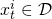is position of theth node and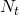total number of nodes;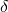denotes the Dirac measure centered on the point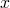. The measure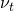describes the distribution of nodes over the space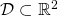of spatial positions. For any node at positionwe define the set of indices of the nodes connected to:The plant grows in a resource landscape. At each time, this resource landscape is represented by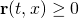the available resources at position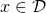. The nodes accessing high levels of resources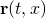are more likely to give birth to new nodes.

### Birth and death rates

Each node ofin positionmay disappear at a death rate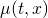and give birth to a new node at a birth rate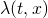. These rates are per capita rates. Global death and birth rates at population level are respectively: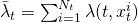and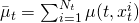; the global event rate is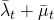. Basically, the per capita rates depend on the local availability of resources: we suppose that the birth rateis an increasing function ofand the death rateis a decreasing function of. When a node is added to the population, it is always linked with the mother node; when a nodeis removed from population, all connections toare suppressed.

### Dispersion kernel

A node at positionat timegives birth to a new node at position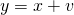according to the p.d.f.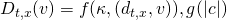.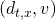is the angle between the preferred direction of reference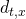and the direction of the new shoot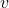.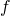is the p.d.f. of the angle of the new shoot and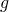is the p.d.f. of the length of the associated link.will be a rough approximation of the gradient of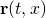given by connected nodes.Dispertion kernel.

### Interactions between nodes and resources

For the coupling of the (discrete) individual dynamics with the resource density dynamicsis modeled as:

(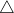)we model with the kernel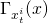the fact that resource consumption is not local.

## Exact Monte Carlo simulation of the IBM

The only approximation is the numerical integration of the resource dynamics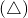which is performed with a finite difference scheme.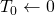,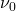,given
for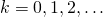do
compute the rate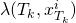and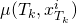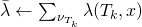,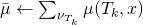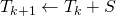with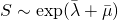if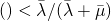then
sampleaccording to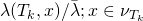sampleaccording to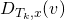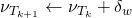[birth]
else
sampleaccording to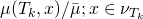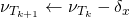end if
compute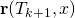[numerical approximation of]
end for

## Simulation

Here the angle p.d.f.is a Von Mises distribution of parameters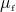and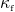; the length p.d.f.is a log-normal distribution of parameters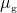and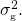The maximum link per node is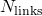.

### Guerrilla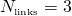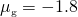,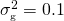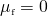,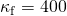:

### Phalanx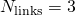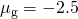,,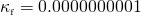: